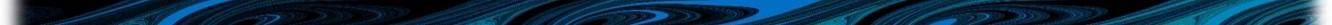# Appendix E Processes: silicate

## E.1 Sediment silicate flux

Silicate is exchanged between the water column and sediments via specification of a sediment flux. In the case of silicate, this flux is most commonly out of the sediments, i.e. a positive specification of sediment flux. Although it is rare that sediments act as sinks of silicate, the WQ Module can be parameterised to allow for this if required.

The user specified rate of sediment silicate flux (which can be spatially varying) is modified by overlying ambient dissolved oxygen concentration, a user specified half saturation oxygen concentration and water temperature. These modifications are simulated via Michaelis-Menten and Arrhenius models, respectively, as per Equation (E.1). $\begin{equation} \href{AppDiags.html#WQDiagSiSedFlx}{F_{sed\langle computed\rangle}^{Si}} = F_{sed}^{Si} \times \underbrace{\frac{K_{sed-O_2}^{Si}}{K_{sed-O_2}^{Si} + \left[DO\right]}}_{\text{Influence of oxygen}} \times \underbrace{\vphantom{\frac{K_{sed-O_2}^{Si}}{K_{sed-O_2}^{Si} + \left[DO\right]}} \left[\theta_{sed}^{Si}\right]^{\left(T-20\right)}}_{\text{Influence of temperature}} \tag{E.1} \end{equation}$ $$F_{sed}^{Si}$$ is the user specified silicate sediment flux at 20$$^o$$C without the influence of dissolved oxygen, $$\left[DO\right]$$ is the overlying dissolved oxygen concentration, $$K_{sed-O_2}^{Si}$$ and $$\theta_{sed}^{Si}$$ are the half saturation concentration of dissolved oxygen and temperature coefficient for silicate sediment production respectively (both are user specified), and $$T$$ is ambient water temperature.

The form of the above relationship between dissolved oxygen concentration and the computed silicate sediment flux, for a range of $$K_{sed-O_2}^{Si}$$ values is provided in E.1. Water temperature is held constant at 20$$^o$$C and $$\theta_{sed}^{Si}$$=1 (i.e. the Arrhenius temperature function is switched off). The user specified benthic silicate sediment flux is 1,120 mg/m$$^2$$/day. Use the “play” button or drag the slider to see how different half saturation oxygen concentrations change the silicate sediment flux (ordinate) with dissolved oxygen concentration (abscissa).

Figure E.1: Move the slider to see the effect of changing the $$K_{sed-O_2}^{Si}$$ value on the computed silicate sediment flux

The figure demonstrates that in this example, the smaller the value of $$K_{sed-O_2}^{Si}$$, the faster the magnitude of the computed silicate sediment flux increases with ambient dissolved oxygen concentration. This is consistent with one interpretation of $$K_{sed-O_2}^{Si}$$, that it is the ambient dissolved oxygen concentration at which the computed silicate sediment flux is half that of the user specified $$F_{sed}^{Si}$$, when temperature effects are turned off.

More generally, for constant dissolved oxygen concentration and $$K_{sed-O_2}^{Si}$$ and without temperature effects, Equation (E.1) has that the computed silicate sediment flux is constant. This means that silicate drawdown also occurs at a constant rate in the demonstration model, so the figure analogous to Figure D.2 is not presented here.

A more realistic environmental setting has sediment silicate fluxes occurring against a background of oxygen concentration drawdown. The demonstration model has been used to illustrate this via execution of a suite of simulations that experience dissolved oxygen drawdown (in the dissolved oxygen constituent model, with $$K_{sed-O_2}^{O_2}$$ = 4 mg/L) and each simulation using a different value of $$K_{sed-O_2}^{Si}$$. The silicate sediment flux rate was specified as above and temperature effects were turned off. The predicted temporal evolution of water column silicate concentration is provided in Figure E.2. Use the “play” button or drag the slider to see how different half saturation concentrations change predicted silicate concentrations (ordinate) in time (abscissa).

Figure E.2: Move the slider to see the effect of changing the $$K_{sed-O_2}^{Si}$$ value on the rate of prodution of silicate in water immediately above the sediments

Finally, the demonstration model was executed over a suite of simulations that had constant sediment flux rates (as above), half saturation coefficients (both $$K_{sed-O_2}^{O_2}$$ and $$K_{sed-O_2}^{Si}$$ = 4 mg/L) and temperature coefficients (both $$\theta_{sed}^{O_2}$$ and $$\theta_{sed}^{Si}$$ = 1.05), but varied ambient water temperature. The predicted temporal evolution of water column silicate concentration is provided in Figure E.3. Use the “play” button or drag the slider to see how different water temperatures change silicate concentrations (ordinate) in time (abscissa).

Figure E.3: Move the slider to see the effect of changing the ambient water temperature on silicate concentration

Although not recommended, the influence of oxygen in all the above processes can be removed by setting the command oxygen == off in the silicate model block.